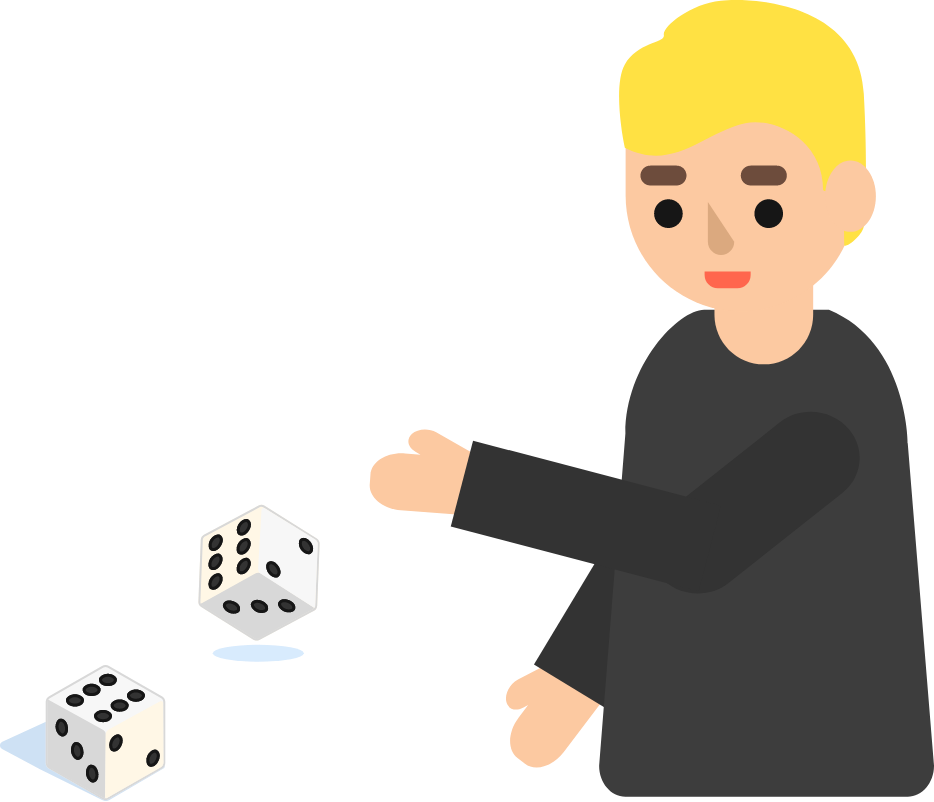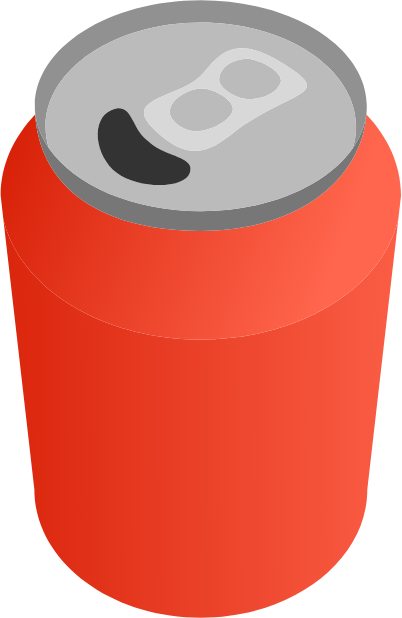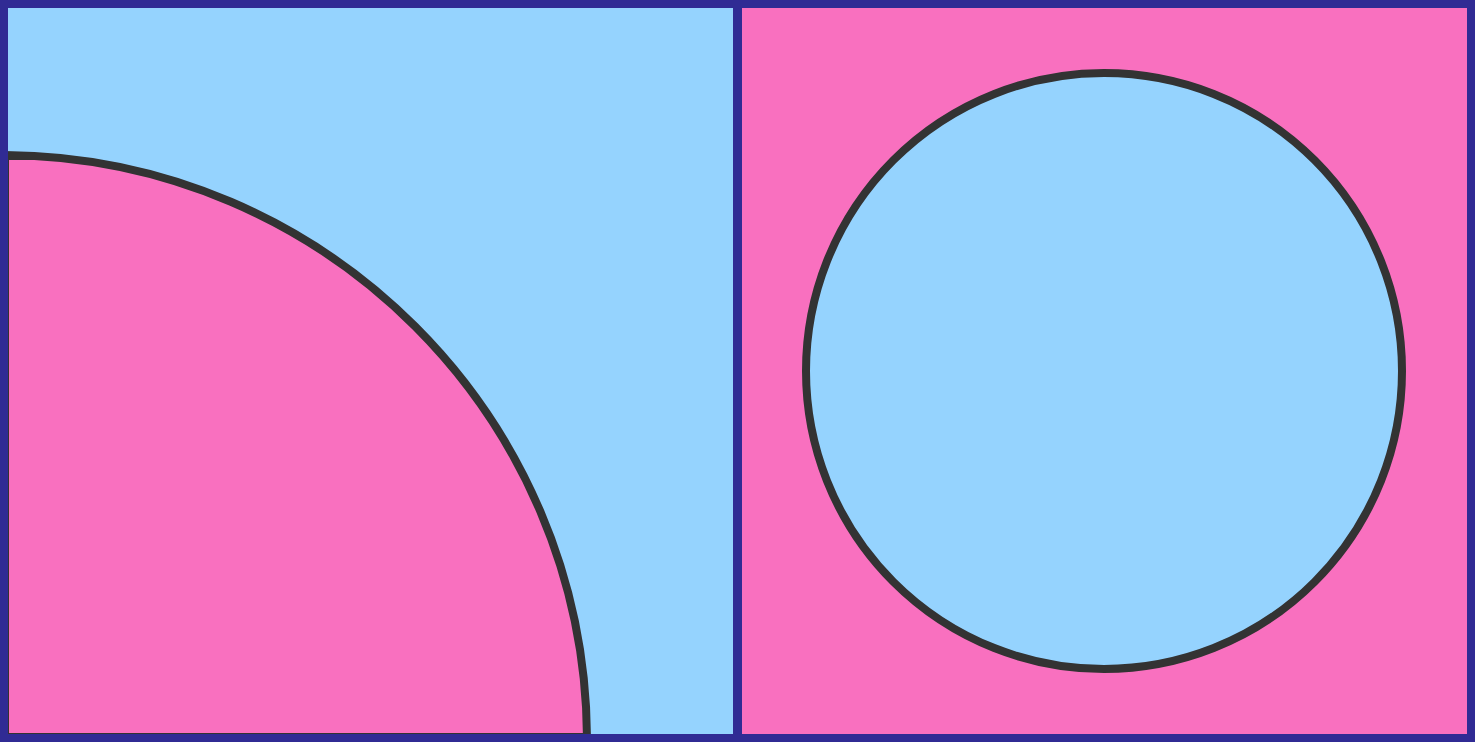# Problems of the Week

Contribute a problem

# 2018-12-10 BasicWhen you roll 2 fair dice, is the total more likely to be odd or even?A full can of soda's center of mass is approximately at its center.

What happens to the center of mass of the (can + soda) system as part of the soda is consumed and the can is placed back on the table, until eventually all of the soda is consumed?

Assume that the soda can is in the shape of a uniform cylinder.

The 2 diagonals of this rectangle divide it into 4 triangles.

If the area of the shaded region is 42, then what is the area of the rectangle?In each square, the blue and red areas are equal.

If $R$ is the radius of the quarter-circle on the left and $r$ is the radius of the circle on the right, what is $\frac{R}{r}?$

I've designed a system which only takes in a prime number as an input and outputs the final digit sum of that number. For example, if I input 997 (a prime), the system will output 7:

$997\ \ \longrightarrow \ \ 9 + 9 + 7 = 25 \ \ \longrightarrow \ \ 2+5=7.$

How many single-digit positive integers cannot be an output of this system?

×